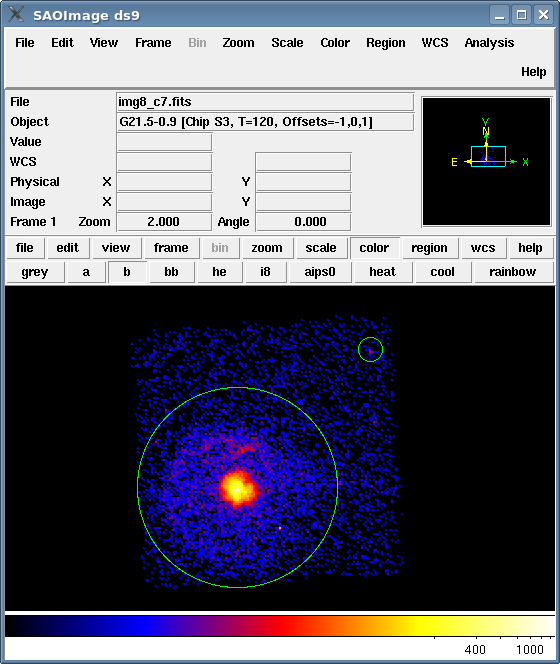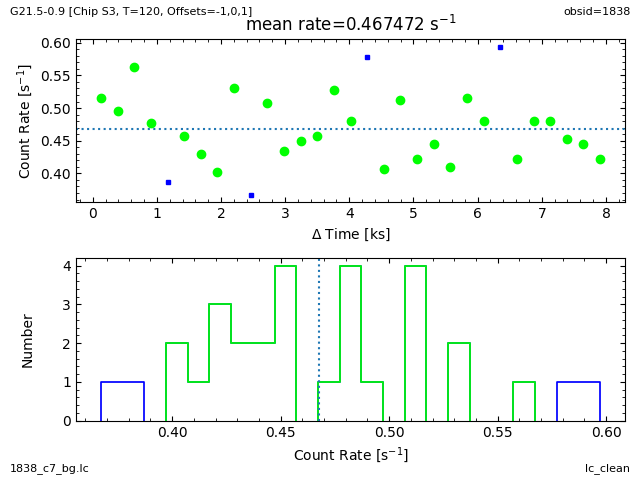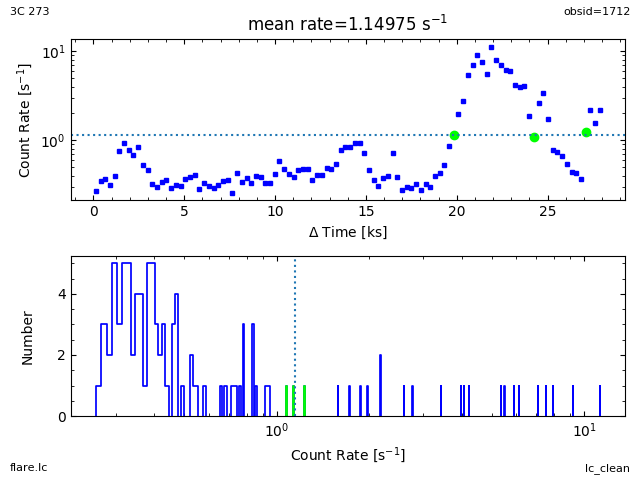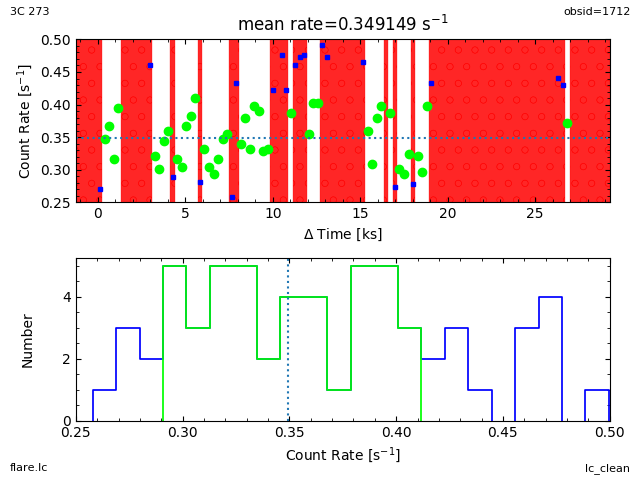# Removing ACIS Background Flares## Overview

#### Synopsis:

This thread may be used to remove background flares from any ACIS dataset.

The ACIS blank-sky background datasets were created for quiescent-background periods. So it is important to filter your dataset to remove periods of background flares before using the blank-sky files in the analysis.

#### Purpose:

To remove background flares from an ACIS observation for spectral fitting or images for spatial analyses.

The lc_clean() routine removes flares (and periods of anomalously-low count rates) from lightcurves using the same technique as used on the ACIS background files. The simple cleaning used by the lc_sigma_clip() routine in the Filtering Lightcurves thread is a more conservative approach.

• The selection of a "true" background can be critical, as discussed in M. Markevitch's memo: astro-ph/0205333.

Last Update: 30 Apr 2019 - Updated for contributed scripts 4.11.2 release with changes to use matplotlib for plotting.

## Get Started

`unix% download_chandra_obsid 1838,1712 evt2,asol`

The observation has been reprocessed to apply the newest gain and CTI calibration. In these examples, we shall restrict attention to the ACIS-S3 chip and the 0.5 to 7 keV energy range:

```unix% dmcopy "acisf01838_repro_evt2.fits[energy=500:7000,ccd_id=7]" 1838_c7.fits
```

ObsID 1712 is used as an example of a strong background flare.

## Remove bright sources

The background fields were filtered using a maximum rate that is 20 percent higher than the quiescent background level. It is therefore necessary to remove any bright sources from your dataset. For this example we have chosen the regions to exclude by eye, using ds9. The resulting region file - sources.reg - is shown on the image in Figure 1 and listed below:

### Figure 1: Source regions overlaid on the image### Figure 1: Source regions overlaid on the image

The regions defined in region file sources.reg overlaid on the image.

```unix% cat sources.reg
# Region file format: CIAO version 1.0
circle(4072.5,4252.5,400)
circle(4604.5,4804.5,48)
```

Now we create a copy of the event file, excluding the sources, using the DM filtering syntax:

```unix% dmcopy "1838_c7.fits[exclude sky=region(sources.reg)]" 1838_c7_bg.fits
```

## Create the lightcurve

The dmextract tool is used to create the lightcurve of the selected region. First, get the GTI interval from the event file:

```unix% dmlist 1838_c7_bg.fits"[GTI7]" data

------------------------------------------------
Data for Table Block GTI7
------------------------------------------------

ROW    START                STOP

1  84245789.1549994648  84253741.1550068706
```

These times will be used as the lower and upper limits for extracting the lightcurve.

The ACIS blank-sky background files were cleaned with a bin size of 259.28 seconds (refer to the Cookbook); the same binning is used for the event data, so the filter is:

```[bin time=84245789.155:84253741.155:259.28]
```

The filter could also be defined as "[bin time=::259.28]", in which case the TSTART and TSTOP header values are used for the endpoints. This approach is valid, though it will probably create some empty bins in the output lightcurve, since that time range is longer than the good time interval.

Run dmextract to create the lightcurve:

```unix% punlearn dmextract
unix% pset dmextract infile="1838_c7_bg.fits[bin time=84245789.155:84253741.155:259.28]"
unix% pset dmextract outfile=1838_c7_bg.lc
unix% pset dmextract opt=ltc1
unix% dmextract
Input event file  (1838_c7_bg.fits[bin time=84245789.155:84253741.155:259.28]):
Enter output file name (1838_c7_bg.lc):
```

The file 1838_c7_bg.lc contains the lightcurve for the data. You can check the parameter file that was used with plist dmextract.

## Remove periods of high (or low) count rates

The lc_clean() routine from the Python module lightcurves.py selects those regions of the lightcurve that do not contain flares. The algorithm used is taken from the lc_clean program created by Maxim Markevitch, and is different from that used by the lc_sigma_clip() routine in the Filtering Lightcurves thread.

The deflare script provides a command-line interface to lc_clean. There is an example of using deflare at the end of this section; see the ahelp file for more information.

The lightcurves module must be loaded into an interactive session of ipython before it can be run (this step is only necessary once per session).

```>>> from lightcurves import *
```

If lc_clean() is called with just the name of the lightcurve file then a diagnostic plot will be produced (Figure 2) and output displayed on the screen:

```>>> lc_clean("1838_c7_bg.lc")
Parameters used to clean the lightcurve are:
script version = February 2019
mean           = None
clip           = 3
scale          = 1.2
minfrac        = 0.1
plot           = True
rateaxis       = y
color          = lime

Total number of bins in lightcurve   = 31
Max length of one bin                = 255.997 s
Num. bins with a smaller exp. time   = 1
Num. bins with exp. time = 0         = 0
Calculated an initial mean (sigma-clipped) rate of 0.469276 ct/s
Filtering lightcurve between rates of 0.391063 and 0.563131 ct/s
Number of good time bins = 27
Rate filter:  0.39106346801286157 <= count_rate < 0.56313139393852063
Mean level of filtered lightcurve = 0.46747244416095812 ct/s

GTI limits calculated using a count-rate filter:
(count_rate>0.39106346801286157 && count_rate<0.56313139393852063)

The corresponding times are:
((time >= 84245789.155000001) && (time < 84246826.275000006)) ; 1.02 ksec, bin 1
((time >= 84247085.555000007) && (time < 84248122.674999997)) ; 1.02 ksec, bin 2
((time >= 84248381.954999998) && (time < 84249937.635000005)) ; 1.54 ksec, bin 3
((time >= 84250196.915000007) && (time < 84252011.875)) ; 1.79 ksec, bin 4
((time >= 84252271.155000001) && (time < 84253826.835000008)) ; 1.45 ksec, bin 5

Exposure time of lightcurve = 7.86 ks
Filtered exposure time      = 6.83 ks
DTCOR value                 = 0.987337

```

### Figure 2: Plot from lc_clean### Figure 2: Plot from lc_clean

The top plot shows the lightcurve of 1838_c7_bg.lc, where the time axis is displayed on the x axis as a relative value (the first bin in the lightcurve is used as the zero point). Points that are in green are those that passed the sigma-clipping and the horizontal dotted line shows the mean value of these points (the numerical value is given in the plot title).

The bottom plot shows a histogram of the count rate values; the green version again shows the data that passes the sigma-clipping algorithm.

In order to create a GTI file, we need to specify an output filename; here we use "1838_c7_bg.gti". The command also creates Figure 3.

```>>> lc_clean("1838_c7_bg.lc", outfile="1838_c7_bg.gti")
Parameters used to clean the lightcurve are:
script version = February 2019
mean           = None
clip           = 3
scale          = 1.2
minfrac        = 0.1
outfile        = 1838_c7_bg.gti
plot           = True
rateaxis       = y
color          = lime
pattern        = solid
pattern color  = red

Total number of bins in lightcurve   = 31
Max length of one bin                = 255.997 s
Num. bins with a smaller exp. time   = 1
Num. bins with exp. time = 0         = 0
Calculated an initial mean (sigma-clipped) rate of 0.469276 ct/s
Filtering lightcurve between rates of 0.391063 and 0.563131 ct/s
Number of good time bins = 27
Rate filter:  0.39106346801286157 <= count_rate < 0.56313139393852063
Mean level of filtered lightcurve = 0.46747244416095812 ct/s

GTI limits calculated using a count-rate filter:
(count_rate>0.39106346801286157 && count_rate<0.56313139393852063)

The corresponding times are:
((time >= 84245789.155000001) && (time < 84246826.275000006)) ; 1.02 ksec, bin 1
((time >= 84247085.555000007) && (time < 84248122.674999997)) ; 1.02 ksec, bin 2
((time >= 84248381.954999998) && (time < 84249937.635000005)) ; 1.54 ksec, bin 3
((time >= 84250196.915000007) && (time < 84252011.875)) ; 1.79 ksec, bin 4
((time >= 84252271.155000001) && (time < 84253826.835000008)) ; 1.45 ksec, bin 5

Exposure time of lightcurve = 7.86 ks
Filtered exposure time      = 6.83 ks
DTCOR value                 = 0.987337

Creating GTI file
Created: 1838_c7_bg.gti
```

### Figure 3: Plot from lc_clean when outfile is set### Figure 3: Plot from lc_clean when outfile is set

The top plot now indicates the regions excluded by the GTI file created by lc_clean - here 1838_c7_bg.gti - using a solid red pattern.

Since we specified an output file, the lc_clean() function has run dmgti, using the calculated range, and created the file 1838_c7_bg.gti. You can check the parameter file that was used with plist dmgti.

### Running lc_clean via the deflare script

The deflare script provides a command-line interface to lc_clean. Run deflare with method=clean to choose the lc_clean cleaning method; here we repeat the example from this section:

```unix% deflare 1838_c7_bg.lc 1838_c7_bg_deflare.gti method=clean \
plot=yes save=deflare_01.png
Parameters used to clean the lightcurve are:
script version = February 2019
mean           = None
clip           = 3
scale          = 1.2
minfrac        = 0.1
outfile        = 1838_c7_bg_deflare.gti
plot           = True
rateaxis       = y
color          = lime
pattern        = solid
pattern color  = red

Total number of bins in lightcurve   = 31
Max length of one bin                = 255.997 s
Num. bins with a smaller exp. time   = 1
Num. bins with exp. time = 0         = 0
Calculated an initial mean (sigma-clipped) rate of 0.469276 ct/s
Filtering lightcurve between rates of 0.391063 and 0.563131 ct/s
Number of good time bins = 27
Rate filter:  0.39106346801286157 <= count_rate < 0.56313139393852063
Mean level of filtered lightcurve = 0.46747244416095812 ct/s

GTI limits calculated using a count-rate filter:
(count_rate>0.39106346801286157 && count_rate<0.56313139393852063)

The corresponding times are:
((time >= 84245789.155000001) && (time < 84246826.275000006)) ; 1.02 ksec, bin 1
((time >= 84247085.555000007) && (time < 84248122.674999997)) ; 1.02 ksec, bin 2
((time >= 84248381.954999998) && (time < 84249937.635000005)) ; 1.54 ksec, bin 3
((time >= 84250196.915000007) && (time < 84252011.875)) ; 1.79 ksec, bin 4
((time >= 84252271.155000001) && (time < 84253826.835000008)) ; 1.45 ksec, bin 5

Exposure time of lightcurve = 7.86 ks
Filtered exposure time      = 6.83 ks
DTCOR value                 = 0.987337

Creating GTI file
Created: 1838_c7_bg_deflare.gti
Light curve cleaned using the lc_clean routine.
Created: deflare_01.png
Press ENTER to close the plot window and exit:
```

To create a plot of the filter, set plot=yes when running deflare.

## Filter the event list

We now filter the event list using the new GTI information, and check to see how the exposure time has changed

```unix% dmcopy "1838_c7.fits[@1838_c7_bg.gti]" 1838_c7_clean.fits

unix% dmkeypar 1838_c7.fits EXPOSURE echo+
7851.3069951925

unix% dmkeypar 1838_c7_clean.fits EXPOSURE echo+
6059.3291110124
```

The new event file, 1838_c7_clean.fits, is ready for use.

The Analysing the ACIS Background with the "Blank-Sky" Files thread shows how to select a background file and match it to the event data.

## An example of a strong flare

The previous example showed an essentially stable background level. ObsID 1712, which is also used in the Filtering Lightcurves, contains a major flare event. First we create a lightcurve of the background of this observation:

```unix% dmcopy "acisf01712N003_evt2.fits[energy=500:7000,ccd_id=7]" 1712_flare.fits

unix% cat exclude.reg
# Region file format: CIAO version 1.0
rotbox(4200.3328,4137.9892,1129.5056,74.07019,24.22333)
circle(4076.5,4088.5,316)
circle(4296.5,5024.5,48)

unix% punlearn dmextract
unix% pset dmextract infile="1712_flare.fits[exclude sky=region(exclude.reg)][bin time=77379634.145:77407432.550:259.28]"
unix% pset dmextract outfile=flare.lc
unix% pset dmextract opt=ltc1
unix% dmextract
Input event file  (1712_flare.fits[exclude sky=region(exclude.reg)][bin time=77379634.145:77407432.550:259.28]):
Enter output file name (flare.lc):
```

When lc_clean() is run on this lightcurve, the routine finds that the filtered lightcurve contains less than ten percent of the original exposure, and so stops (this limit can be changed by setting the option minfrac to something other than 0.1). The diagnostic plot that is created (Figure 4) shows that the default options do not do a good job with this lightcurve. The log_scale calls are used to change the scaling of the rate axes in both plots.

```>>> lc_clean("flare.lc")
Parameters used to clean the lightcurve are:
script version = February 2019
mean           = None
clip           = 3
scale          = 1.2
minfrac        = 0.1
plot           = True
rateaxis       = y
color          = lime

Total number of bins in lightcurve   = 108
Max length of one bin                = 255.997 s
Num. bins with a smaller exp. time   = 1
Num. bins with exp. time = 0         = 0
Calculated an initial mean (sigma-clipped) rate of 1.23439 ct/s
Filtering lightcurve between rates of 1.02866 and 1.48127 ct/s
Number of good time bins = 3
Rate filter:  1.0286585287415402 <= count_rate < 1.4812682813878177
Mean level of filtered lightcurve = 1.1497537732642782 ct/s

Warning: Default bin width of 0.01 count/s is too small as it produces
1099.2323164096306 bins.
Replacing with a width of 0.0109923 count/s
This may indicate that the lightcurve contains strong flares that
require manual filtering.

Fraction of bins that are good (0.0277778, 3 of 108) is below limit of 0.1!

>>> import matplotlib.pylab as plt
>>> plt.xscale("log")
>>> plt.gcf().axes.set_yscale("log")  # change top plot

```

### Figure 4: A lightcurve with a strong flare in it### Figure 4: A lightcurve with a strong flare in it

The strong flare means that the routine does not pick up the "quiescent" level in this obeservation. Since the X axis of the bottom plot is linked to the Y axis of the top plot, the log_scale has changed the scaling in both plots (e.g. compare to Figure 2).

The rate histogram in Figure 4 suggests a value of 0.35 count/s, so we use the mean option to force this value:

```>>> lc_clean("flare.lc", mean=0.35, outfile="flare.gti")
Parameters used to clean the lightcurve are:
script version = February 2019
mean           = 0.35
clip           = 3
scale          = 1.2
minfrac        = 0.1
outfile        = flare.gti
plot           = True
rateaxis       = y
color          = lime
pattern        = solid
pattern color  = red

Total number of bins in lightcurve   = 108
Max length of one bin                = 255.997 s
Num. bins with a smaller exp. time   = 1
Num. bins with exp. time = 0         = 0
Using a fixed mean rate of 0.35 ct/s
Filtering lightcurve between rates of 0.291667 and 0.42 ct/s
Number of good time bins = 42
Rate filter:  0.2916666666666667 <= count_rate < 0.42
Mean level of filtered lightcurve = 0.34914865433053721 ct/s

Warning: Default bin width of 0.01 count/s is too small as it produces
1099.2323164096306 bins.
Replacing with a width of 0.0109923 count/s
This may indicate that the lightcurve contains strong flares that
require manual filtering.

GTI limits calculated using a count-rate filter:
(count_rate>0.2916666666666667 && count_rate<0.42)

The corresponding times are:
((time >= 77379893.424999997) && (time < 77380930.545000002)) ; 1.02 ksec, bin 1
((time >= 77382745.504999995) && (time < 77383782.625)) ; 1.02 ksec, bin 2
((time >= 77384041.905000001) && (time < 77385338.304999992)) ; 1.28 ksec, bin 3
((time >= 77385597.584999993) && (time < 77387153.265000001)) ; 1.54 ksec, bin 4
((time >= 77387671.825000003) && (time < 77389486.784999996)) ; 1.79 ksec, bin 5
((time >= 77390523.905000001) && (time < 77390783.185000002)) ; 0.26 ksec, bin 6
((time >= 77391561.024999991) && (time < 77392338.864999995)) ; 0.77 ksec, bin 7
((time >= 77394931.664999992) && (time < 77395968.784999996)) ; 1.02 ksec, bin 8
((time >= 77396228.064999998) && (time < 77396487.344999999)) ; 0.26 ksec, bin 9
((time >= 77396746.625) && (time < 77397524.465000004)) ; 0.77 ksec, bin 10
((time >= 77397783.74499999) && (time < 77398561.584999993)) ; 0.77 ksec, bin 11
((time >= 77406339.984999999) && (time < 77406599.265000001)) ; 0.26 ksec, bin 12

Exposure time of lightcurve = 27.45 ks
Filtered exposure time      = 10.75 ks
DTCOR value                 = 0.987337

Creating GTI file
Created: flare.gti
```

The resulting plot (Figure 5) shows that more data is included but that the data is still noisy, which suggests that care probably needs to be taken in interpreting the results.

### Figure 5: Setting the mean option when filtering a lightcurve### Figure 5: Setting the mean option when filtering a lightcurve

Forcing the initial calculation to use a mean level of 0.35 count/s has resulted in more points being marked as good. However, the curve is still noisy and many times are excluded, as indicated by the regions of solid red color.

The use of AUTO for the minimum value in the limits call means that the limit is calculated from the data.

See the lc_clean help file for more information on changing the filtering scheme used by the routine. Maxim Markevitch's Cookbook contains advice on filtering lightcurves with strong flares.

```

#--------------------------------------------------------------------
#
# DMEXTRACT -- extract columns or counts from an event list
#
#--------------------------------------------------------------------
infile = 1838_c7_bg.fits[bin time=84245789.155:84253741.155:259.28] Input event file
outfile = 1838_c7_bg.lc    Enter output file name
(bkg = )                Background region file or fixed background (counts/pixel/s) subtraction
(error = gaussian)        Method for error determination(poisson|gaussian|<variance file>)
(bkgerror = gaussian)        Method for background error determination(poisson|gaussian|<variance file>)
(bkgnorm = 1.0)             Background normalization
(exp = )                Exposure map image file
(bkgexp = )                Background exposure map image file
(sys_err = 0)               Fixed systematic error value for SYS_ERR keyword
(opt = ltc1)            Output file type: pha1
(defaults = \${ASCDS_CALIB}/cxo.mdb -> /soft/ciao/data/cxo.mdb) Instrument defaults file
(wmap = )                WMAP filter/binning (e.g. det=8 or default)
(clobber = no)              OK to overwrite existing output file(s)?
(verbose = 0)               Verbosity level
(mode = ql)
```

```

infile = 1838_c7_bg.lc    Input MTL file
outfile = 1838_c7_bg.gti   Output GTI file
userlimit = (count_rate>0.22473510778229128 && count_rate<0.3236185552064994) User defined limit string
(mtlfile = none)            Optional output smoothed/filtered MTL file
(lkupfile = none)            Lookup table defining which MTL columns to check against (NONE|none|<filename>)
(smooth = yes)             Smooth the input MTL data?
(clobber = yes)             Clobber output file if it exists?
(verbose = 0)               Debug level
(mode = h)
```

## History

 24 Jan 2012 new for CIAO 4.4: previously was a section in the Analysing the ACIS Background with the "Blank-Sky" Files thread 03 Dec 2012 Review for CIAO 4.5; no changes. 02 Dec 2013 Review for CIAO 4.6; minor edits 17 Dec 2014 Review for CIAO 4.7; no changes. 30 Apr 2019 Updated for contributed scripts 4.11.2 release with changes to use matplotlib for plotting.